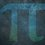# UKMT Special (Problem $16$)

Find all real numbers $x, y, z$ which satisfy the simultaneous equations

$x^2 - 4y + 7 = 0$

$y^2 - 6z + 14 = 0$

$z^2 - 2x - 7 = 0$

[UKMT BMO Round $1$ $2012$, Q$3$]Note by Yajat Shamji
8 months, 2 weeks ago

This discussion board is a place to discuss our Daily Challenges and the math and science related to those challenges. Explanations are more than just a solution — they should explain the steps and thinking strategies that you used to obtain the solution. Comments should further the discussion of math and science.

When posting on Brilliant:

• Use the emojis to react to an explanation, whether you're congratulating a job well done , or just really confused .
• Ask specific questions about the challenge or the steps in somebody's explanation. Well-posed questions can add a lot to the discussion, but posting "I don't understand!" doesn't help anyone.
• Try to contribute something new to the discussion, whether it is an extension, generalization or other idea related to the challenge.

MarkdownAppears as
*italics* or _italics_ italics
**bold** or __bold__ bold
- bulleted- list
• bulleted
• list
1. numbered2. list
1. numbered
2. list
Note: you must add a full line of space before and after lists for them to show up correctly
paragraph 1paragraph 2

paragraph 1

paragraph 2

[example link](https://brilliant.org)example link
> This is a quote
This is a quote
    # I indented these lines
# 4 spaces, and now they show
# up as a code block.

print "hello world"
# I indented these lines
# 4 spaces, and now they show
# up as a code block.

print "hello world"
MathAppears as
Remember to wrap math in $$ ... $$ or $ ... $ to ensure proper formatting.
2 \times 3 $2 \times 3$
2^{34} $2^{34}$
a_{i-1} $a_{i-1}$
\frac{2}{3} $\frac{2}{3}$
\sqrt{2} $\sqrt{2}$
\sum_{i=1}^3 $\sum_{i=1}^3$
\sin \theta $\sin \theta$
\boxed{123} $\boxed{123}$

Sort by:

You have until next Friday, $3:00$pm!

- 8 months, 2 weeks ago

Step 1) Add all of them and factor the term.

   x^2 - 2x + y^{2} - 4y + z^{2} - 6z + 14 = 0

x^2 - 2x + 1 + y^2 - 4y + 4 + z^2 - 6z + 9 = 0

(x - 1)^2 + (y - 2)^2 + (z - 3)^2 = 0


Step 2) If any of the squared term is not 0, it must be a positive real number.

If there is at least one squared term that is not 0, the equation will be false because the right hand sight must be greater than 0.

So, each the square term must be equal to 0.

   (x - 1)^2 = 0    =>    x - 1  = 0    =>    x=1

(y - 2)^2 = 0    =>    y - 2 = 0    =>    y=2

(z - 3)^2 = 0    =>    z - 3 = 0    =>    z=3


(x , y , z)=(1 , 2 , 3) is the only real solution.

I apologize for the bad representation.

- 8 months, 2 weeks ago

Correct method and solution!

- 8 months, 2 weeks ago